1600718400

# PyTorch for Deep Learning — AutoGrad and Simple Linear Regression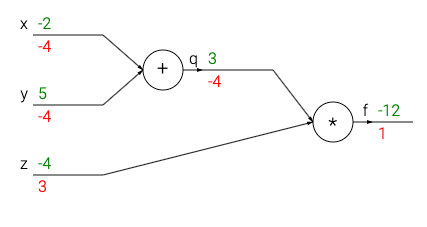PyTorch’s AutoGrad is a very powerful feature with which we can easily find the differentiation of a variable with respect to another. This comes handy while calculating gradients for gradient descent algorithm

## How to use this feature

first and foremost , let’s import the necessary libraries

``````#importing the libraries

import torch
import numpy as np
import matplotlib.pyplot as plt
import random
x = torch.tensor(5.) #some data
w = torch.tensor(4.,requires_grad=True) #weight ( slope )
y = x*w + b #equation of a line
y.backward() #letting pytorch know that Y is the variable that needs to be differentiated
print(w.grad,b.grad) #prints the derivative of Y with respect to w and b
output:
tensor(5.) tensor(1.)
``````

This is the basic idea behind PyTorch’s AutoGrad.

the** backward()** function specify the variable to be differentiated

and the .grad prints the differentiation of that function with respect to the variable.

## Buddha Community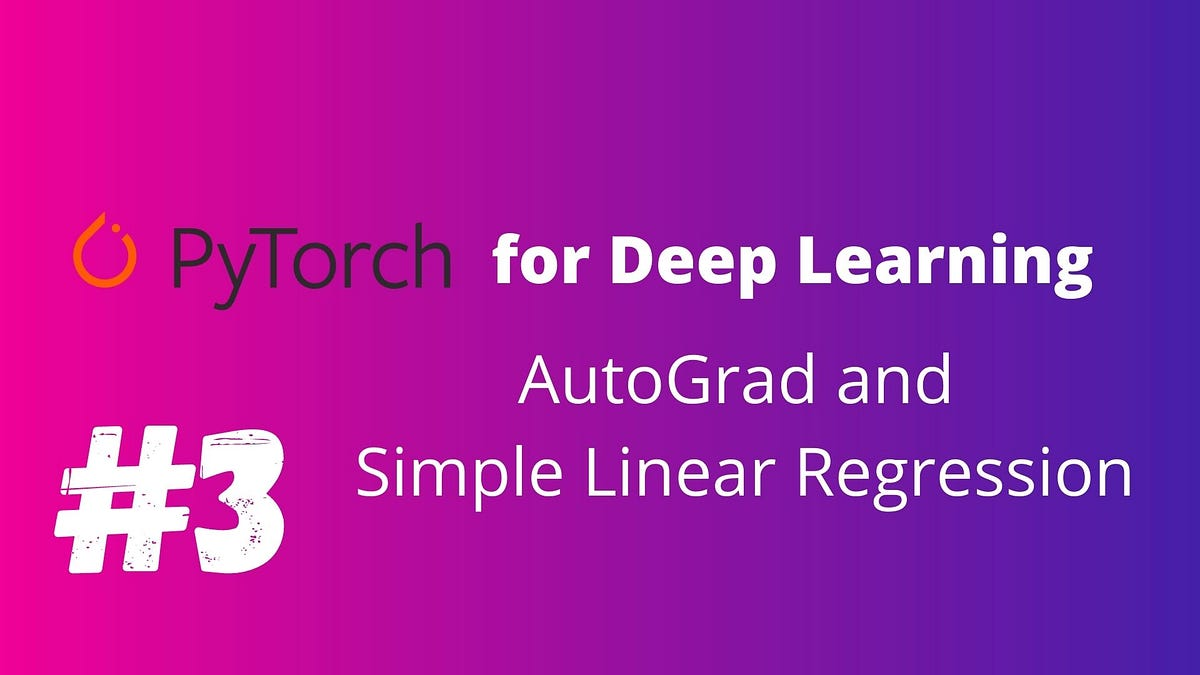1600718400

## PyTorch for Deep Learning — AutoGrad and Simple Linear RegressionPyTorch’s AutoGrad is a very powerful feature with which we can easily find the differentiation of a variable with respect to another. This comes handy while calculating gradients for gradient descent algorithm

## How to use this feature

first and foremost , let’s import the necessary libraries

``````#importing the libraries

import torch
import numpy as np
import matplotlib.pyplot as plt
import random
x = torch.tensor(5.) #some data
w = torch.tensor(4.,requires_grad=True) #weight ( slope )
y = x*w + b #equation of a line
y.backward() #letting pytorch know that Y is the variable that needs to be differentiated
print(w.grad,b.grad) #prints the derivative of Y with respect to w and b
output:
tensor(5.) tensor(1.)
``````

This is the basic idea behind PyTorch’s AutoGrad.

the** backward()** function specify the variable to be differentiated

and the .grad prints the differentiation of that function with respect to the variable.1618317562

## Top Deep Learning Development Services | Hire Deep Learning Developer

We at Inexture, strategically work on every project we are associated with. We propose a robust set of AI, ML, and DL consulting services. Our virtuoso team of data scientists and developers meticulously work on every project and add a personalized touch to it. Because we keep our clientele aware of everything being done associated with their project so there’s a sense of transparency being maintained. Leverage our services for your next AI project for end-to-end optimum services.

#deep learning development #deep learning framework #deep learning expert #deep learning ai #deep learning services1597277100

## Linear Regression in PyTorch

### A brief introduction to Linear Regression in PyTorch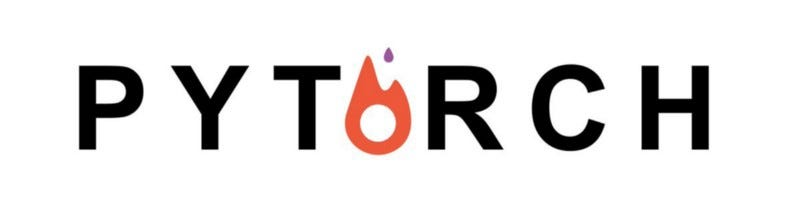For all those amateur Machine Learning and Deep Learning enthusiasts out there, Linear Regression is just the right way to kick start your journey. If you are new to Machine Learning with some background in PyTorch, then buckle up because you have just ended up at the right spot. In case you are not, don’t worry, checkout my previous article to pick up some fundamentals of PyTorch and then you are all good to go.

## So what is Linear Regression ?

Linear Regression is a linear model that predicts the output, given a set of input variables, assuming that there is a linear relationship between the input variables and the single output variable.

For instance let’s just consider the simple linear equation y = w*x + b. Here y is the output and x is the input variable. “w” and “b” forms the slope and y intercept of the equation respectively. So we will refer to “w” and “b” as the parameters of the equation because once you get to know these values, you can easily predict the output for a given value of x.

Now let’s slightly change the scenario. Assume that you are given the values of x and y (we will call them the training set ) and you are asked to find out the new value of y corresponding to a new variable x. Obviously, simple linear algebra would do the trick and easily give you the right parameters . Once you plug them into the equation you can find out the new value of y, corresponding to x, in no time.

So what’s the big deal, these are things that we have covered in our high school. But here is what you should consider:

1. Real world datasets may have noise in them i.e. there may be some values of x and y, in your training set, that would not go hand in hand with some others values of x and y in the same set, obviously putting an end to your attempt to find out the right values of the parameters using the traditional approach.
2. Also in real world datasets there may be more than one variable determining an output variable thus it becomes a hefty task to find out the parameters when several input variables are involved.

This is where Machine learning steps in. I will try to give you an overview of what is happening behind the scenes of the infamous Linear regression method. Initially our Machine know nothing more than an average child. It will take some random values of “w” and “b”, which from now on we will refer to as the weight and the bias, and plug those into the equation y = w*x + b. Now it will take some values of x in the training set and find out the corresponding values of y using the parameters that it had assumed earlier. It will then compare the predicted values of y say yhat, with the actual values of y by calculating something known as the loss function (cost function).

The loss function essentially denotes the error in our prediction. A greater value of the loss function denotes a greater error. Here we will be using Mean Square Error (MSE) as our loss function and it is given by the formula :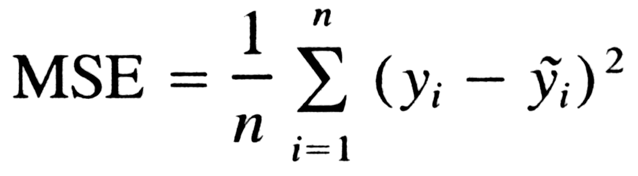Once the loss is calculated the we perform an optimization method like gradient descent on the loss function. Stuck with gradient descent, don’t worry, for the time sake all you have to know is that gradient descent is simply a method performed on the loss function to find the values of “w” and “b” that minimizes the loss function. It involves the use of learning rate which can be tweaked for better results. Smaller the loss more accurate is our prediction.

#deep-learning #pytorch #linear-regression #machine-learning #deep learning1595571540

## Chapter 4.2 — Linear Regression using PyTorch Built-ins

In last blog Chapter 4.1 we discussed in detail about some commonly used built-in PyTorch packages and some basic concepts we will be using to build out linear regression model. In this blog we will be building our model using the PyTorch built-ins.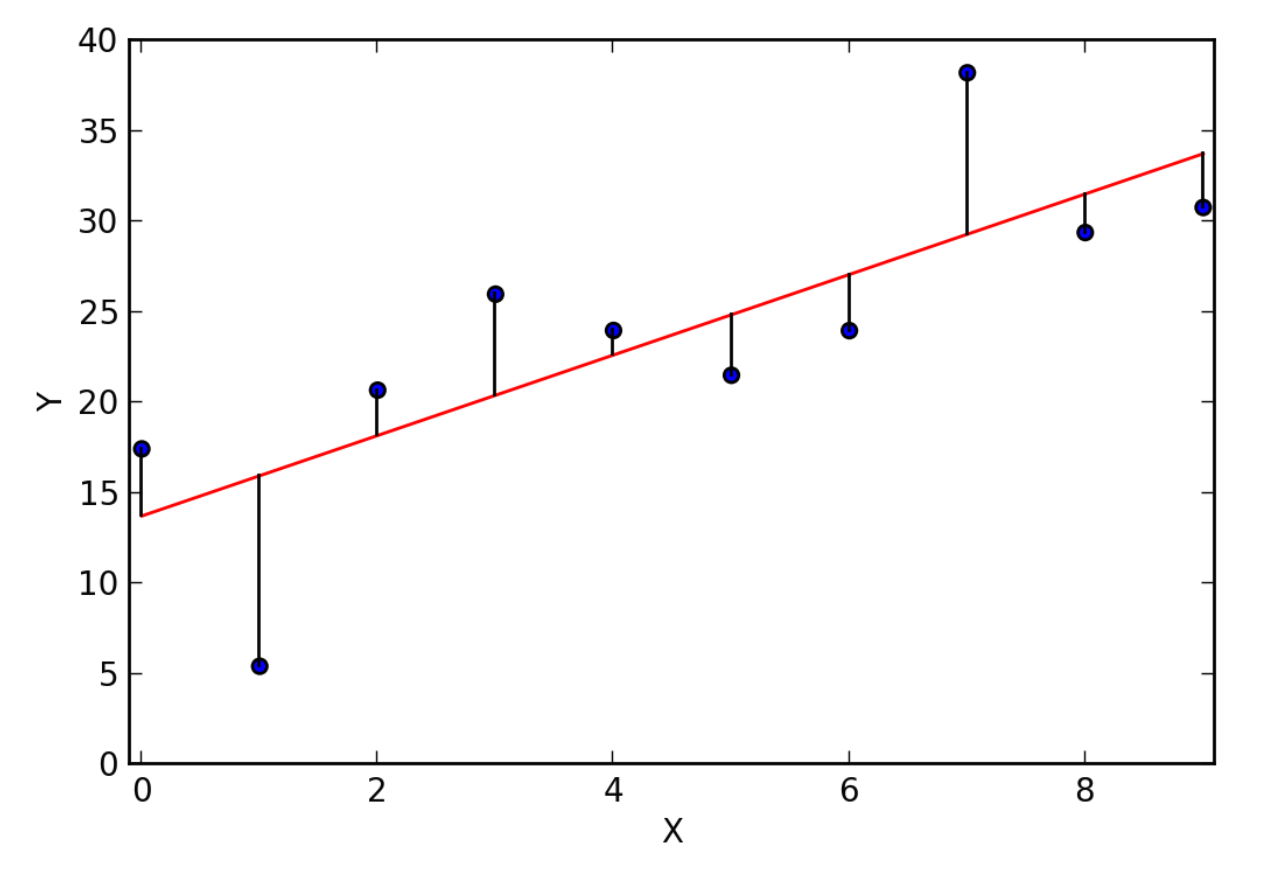In this blog, we’re going to use information like a person’s age, sex, BMI, no. of children and smoking habit to accurately predict insurance costs. This kind of model is useful for insurance companies to determine the yearly insurance premium for a person. The dataset for this problem is taken from: .

We will create a model with the following steps:

2. Prepare the dataset for training ,
3. Create a linear regression model ,
4. Train the model to fit the data ,
5. Make predictions using the trained model.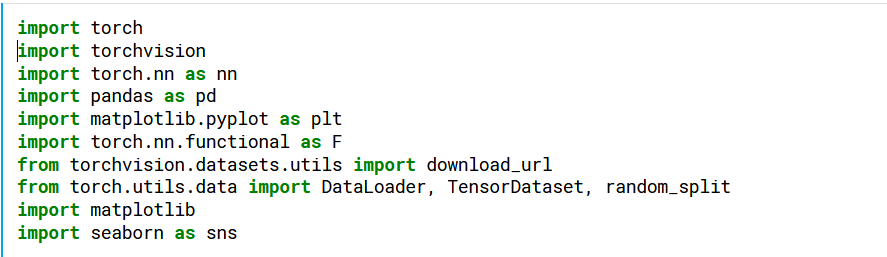Generated By Author

We start by importing the required packages. We have discussed about most of the packages used in the previous blog.

For this blog, we will be using Kaggle platform to build our model. We could load our dataset directly from Kaggle.

To load the dataset into memory, we’ll use the read_csv function from the pandas library. The data will be loaded as a Pandas dataframe.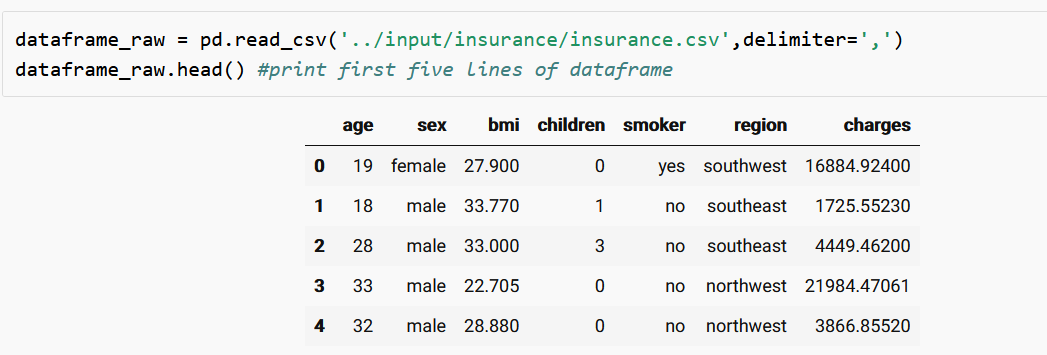Generated by Author

We could print the first five lines of the dataset using the head function in Pandas.

We are going to do a slight customization to dataset so that every reader could get a slightly different dataset. This step is not mandatory.Generated By Author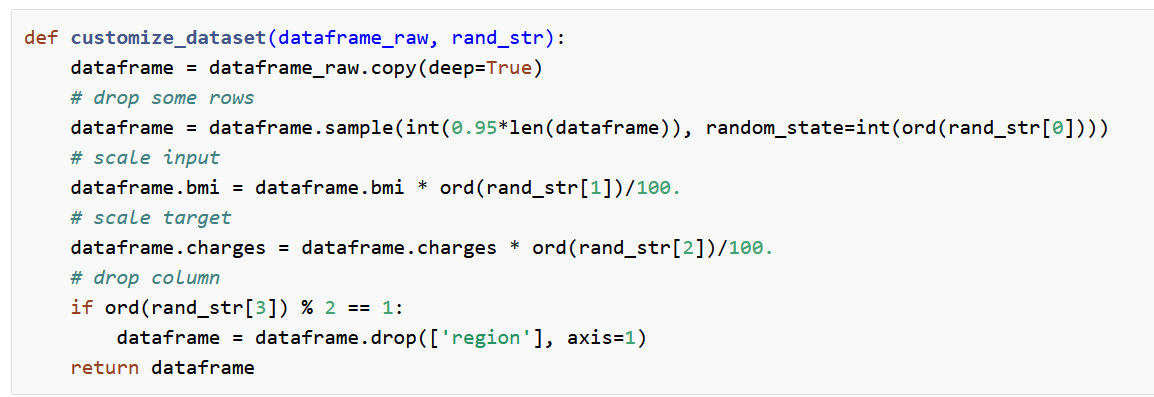Generated by Author

The customize_dataset function will customize the dataset slightly using your name as a source of random numbers.

Now let’s call the customize function and pass dataset and your_name as arguments and check out first few lines of our dataset using the head function.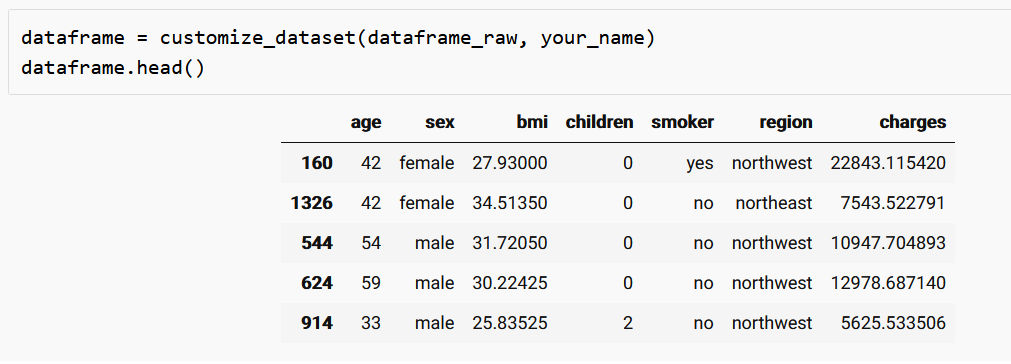Generated by Author

Now let’s find out the number of rows and columns in our dataset.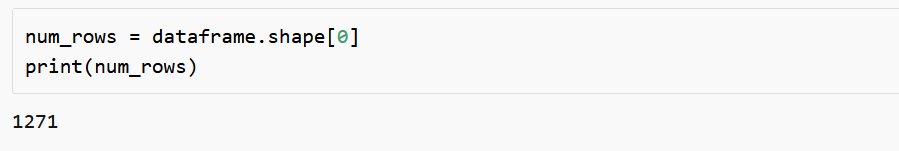Generated By Author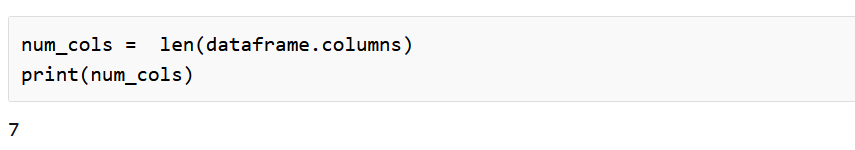Generated by Author

Now we should assign the input, output and categorical columns(input columns that are non-numerical).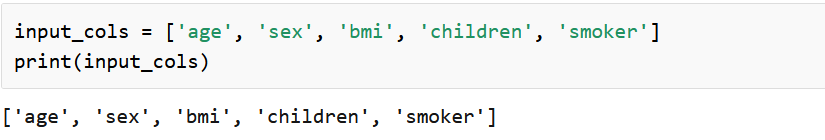Generated by Author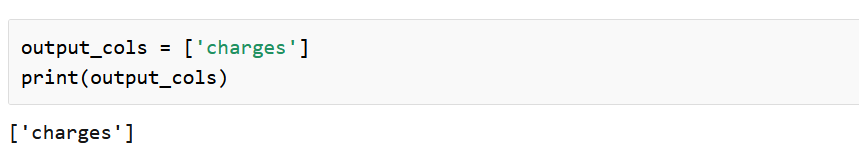Generated by Author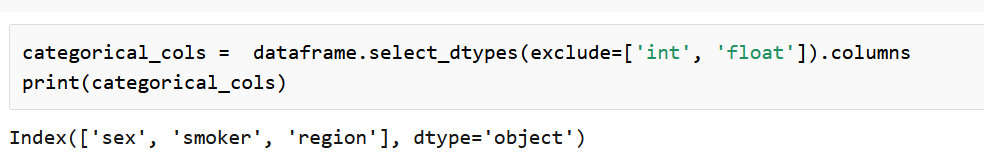Generated by Author

We can find the minimum value, maximum value and mean value of output column “charges”. We can also plot the distribution of charges in a graph. For reference do look into https://jovian.ml/aakashns/dataviz-cheatsheet.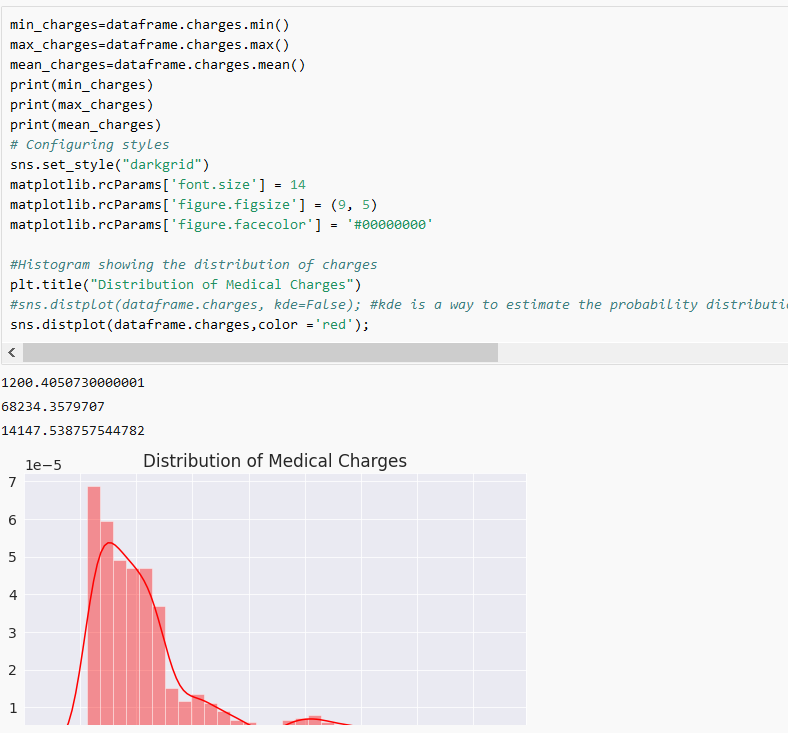Generated by Author

#linear-regression #deep-learning #machine-learning #visualization #pytorch #deep learning1596327780

## Linear regression is a common machine learning technique that predicts a real-valued output using a weighted linear combination of one or more input values.

The “learning” part of linear regression is to figure out a set of weights w1, w2, w3, … w_n, b that leads to good predictions. This is done by looking at lots of examples one by one (or in batches) and adjusting the weights slightly each time to make better predictions, using an optimisation technique called Gradient Descent.

Let’s create some sample data with one feature “x” and one dependent variable “y”. We’ll assume that “y” is a linear function of “x”, with some noise added to account for features we haven’t considered here. Here’s how we generate the data points, or samples:

And here’s what it looks like visually:

Now we can define and instantiate a linear regression model in PyTorch:

# Loss function

Machines learn by means of a loss function. It’s a method of evaluating how well specific algorithm models the given data. Here we will use Mean Square Error

We will use Stochastic Gradient Descent as our optimiser.

Finally… now we will train our model and visualise our linear regression model being trained.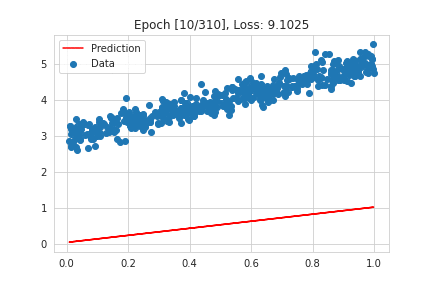#artificial-intelligence #deep-learning #linear-regression #pytorch #machine-learning #deep learning